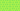PricingResources

# What Are Quartiles?

Written by

There are many statistical formulas which can be useful for analysing data. One of these is the quartile formula, used to divide data points or groups of observations. Keep reading to learn what this means, as well as how to calculate quartiles.

A quartile is a term used in statistics. As you might guess from the name, it’s used to divide a data set into four groups, also called intervals. These four intervals are based both on the data values, as well as how they relate to the entire set.

While data points can be dotted all over a graph at random, to organise them into quartiles you’ll need to plot them on a number line. They’re listed in ascending order and then divided into four quarters. Quartiles are quite similar to a median, simply dividing the data into four equal parts rather than two.

## How do quartiles work?

To better visualise the purpose of calculating quartiles, it’s helpful to explain the median. The median is the middle value of any set of numbers. It represents the point at which half of the data points lie above and below the central number, or median.

If you imagine the numbers 1-13 set out on a number line, number 7 would be the median as it sits directly in the middle. Half of the values are higher than 7, and half of the values are lower than 7.

Quartiles take this idea of central tendency a step further. Calculating the median of a data set has some limits, because it doesn’t tell you anything about the spread of the numbers on either side. A quartile measures the spread or distribution of values both above and below the middle point.

Here’s the breakdown of each quartile:

• Lower quartile (Q1): The lowest 25% of data is separated from the highest 75%. It represents the middle number between the median and the dataset’s smallest value.

• Middle quartiles (Q2): These are the same as the median of the data set, including the two middle sections of the data set – 50% of the data is less than the mean.

• Upper quartile (Q3): This distinguishes the highest 25% of data points from the lowest 75%. It’s the central point between the median and the highest number of the set.

Using this breakdown, you can map out the four intervals or groups gleaned from these quartiles.

• Interval 1: Smallest value up to Q1

• Interval 2: Q1 up to median

• Interval 3: Median to Q3

• Interval 4: Q3 to highest value

In this way, each quartile or interval includes 25% of the total data or observations, arranged from smallest value to largest.

## How to calculate quartiles

For smaller data sets, it’s simple to work out quartiles yourself. Imagine that you have a data set with N values or data points.

Taking this data set, here is what the quartile formula looks like:

• Lower Quartile (Q1) = (N+1) * 1/4

• Middle Quartile (Q2) = (N+1) * 2/4

• Upper Quartile (Q3) = (N+1) * 3/4

## QUARTILE function in Excel

If you need to apply the quartile formula to larger data sets, it’s easiest to use the QUARTILE function in Microsoft Excel. It’s listed under Statistical Functions. All you need to do is enter the set of values into the box and it will return the quartile. You can also plug the QUARTILE function into any cell in your worksheet as part of a formula, and it will return the minimum value, first, second and third quartile, and maximum value.

So, what does this all mean for financial analysis? Calculating quartiles is useful because it helps you break down things like revenues over time or inventory sales. In the real world, the quartile formula breaks down large data sets into four manageable pieces, for better understanding of their relevance.

#### We can help

GoCardless helps you automate payment collection, cutting down on the amount of admin your team needs to deal with when chasing invoices. Find out how GoCardless can help you with ad hoc payments or recurring payments.

Sales

Support

+61 3 8375 9198